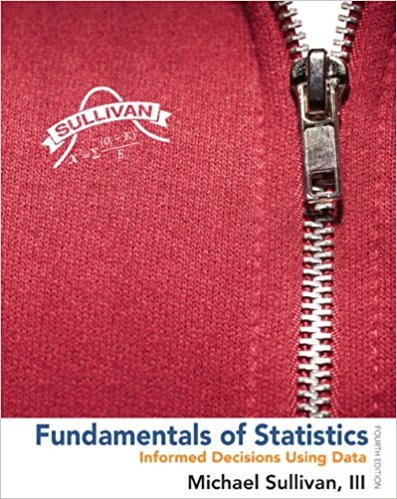×
×

# Solutions for Chapter Chapter 7.3: Fundamentals of Statistics 4th Edition## Full solutions for Fundamentals of Statistics | 4th Edition

ISBN: 9780321838704Solutions for Chapter Chapter 7.3

Solutions for Chapter Chapter 7.3
4 5 0 362 Reviews
30
2
##### ISBN: 9780321838704

Chapter Chapter 7.3 includes 14 full step-by-step solutions. Fundamentals of Statistics was written by and is associated to the ISBN: 9780321838704. Since 14 problems in chapter Chapter 7.3 have been answered, more than 284432 students have viewed full step-by-step solutions from this chapter. This textbook survival guide was created for the textbook: Fundamentals of Statistics, edition: 4. This expansive textbook survival guide covers the following chapters and their solutions.

Key Statistics Terms and definitions covered in this textbook
• Alternative hypothesis

In statistical hypothesis testing, this is a hypothesis other than the one that is being tested. The alternative hypothesis contains feasible conditions, whereas the null hypothesis speciies conditions that are under test

• Attribute

A qualitative characteristic of an item or unit, usually arising in quality control. For example, classifying production units as defective or nondefective results in attributes data.

• Attribute control chart

Any control chart for a discrete random variable. See Variables control chart.

• Bimodal distribution.

A distribution with two modes

• Cause-and-effect diagram

A chart used to organize the various potential causes of a problem. Also called a ishbone diagram.

• Center line

A horizontal line on a control chart at the value that estimates the mean of the statistic plotted on the chart. See Control chart.

• Combination.

A subset selected without replacement from a set used to determine the number of outcomes in events and sample spaces.

• Continuity correction.

A correction factor used to improve the approximation to binomial probabilities from a normal distribution.

• Contour plot

A two-dimensional graphic used for a bivariate probability density function that displays curves for which the probability density function is constant.

• Correction factor

A term used for the quantity ( / )( ) 1 1 2 n xi i n ? = that is subtracted from xi i n 2 ? =1 to give the corrected sum of squares deined as (/ ) ( ) 1 1 2 n xx i x i n ? = i ? . The correction factor can also be written as nx 2 .

• Correlation

In the most general usage, a measure of the interdependence among data. The concept may include more than two variables. The term is most commonly used in a narrow sense to express the relationship between quantitative variables or ranks.

• Covariance matrix

A square matrix that contains the variances and covariances among a set of random variables, say, X1 , X X 2 k , , … . The main diagonal elements of the matrix are the variances of the random variables and the off-diagonal elements are the covariances between Xi and Xj . Also called the variance-covariance matrix. When the random variables are standardized to have unit variances, the covariance matrix becomes the correlation matrix.

• Critical region

In hypothesis testing, this is the portion of the sample space of a test statistic that will lead to rejection of the null hypothesis.

• Cumulative distribution function

For a random variable X, the function of X deined as PX x ( ) ? that is used to specify the probability distribution.

• Defect concentration diagram

A quality tool that graphically shows the location of defects on a part or in a process.

• Density function

Another name for a probability density function

• Error mean square

The error sum of squares divided by its number of degrees of freedom.

• Error propagation

An analysis of how the variance of the random variable that represents that output of a system depends on the variances of the inputs. A formula exists when the output is a linear function of the inputs and the formula is simpliied if the inputs are assumed to be independent.

• Fixed factor (or fixed effect).

In analysis of variance, a factor or effect is considered ixed if all the levels of interest for that factor are included in the experiment. Conclusions are then valid about this set of levels only, although when the factor is quantitative, it is customary to it a model to the data for interpolating between these levels.

• Gamma random variable

A random variable that generalizes an Erlang random variable to noninteger values of the parameter r

×